## Clifford's Circle Theorem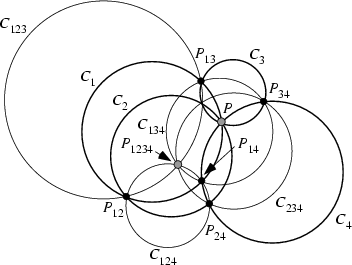Let,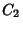,, andbe four Circles of General Position through a point. Letbe the second intersection of the Circlesand. Letbe the Circle. Then the four Circles,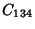,, andall pass through the point. Similarly, let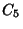be a fifth Circle through. Then the five points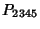,,,andall lie on one Circle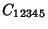. And so on.